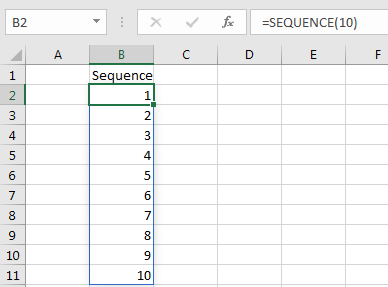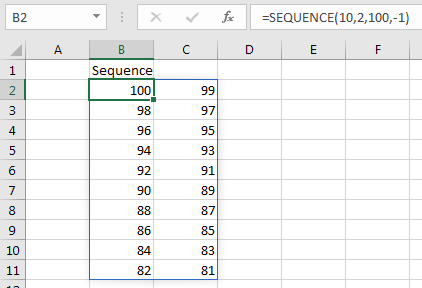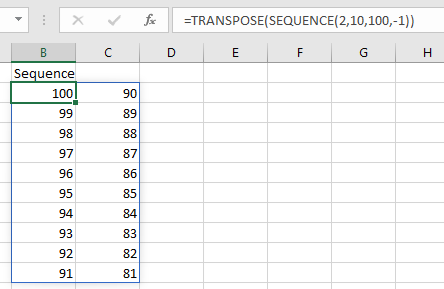# The SEQUENCE Function in Excel

To get a sequence of numbers we used to use a combination of functions but now MS has provided one function for getting sequential numbers.
The SEQUENCE function of Excel returns a sequence of numeric values. You can also adjust the steps in the sequence along with the rows and columns.

Syntax of the SEQUENCE function

=SEQUENCE(rows,[columns],[start],[step])

Rows: The number of rows to which you want to spill the sequence.

[column]: The number of columns to which you want to spill the sequence. The numbers will first fill in the columns and then rows. The column is optional. By default, it is 1.

[start]: Optional. The starting number of the sequence. By default, it is 1.

[step]: This the increment number for the next number. By default, it is 1.

Let's have some examples to learn how we can use the SEQUENCE function in Excel.

Example1: Get Sequence of 1 to 10 in Range B2:B11

So, the task is simple. We just need to get the sequential numbers in a range using a formula. It is only simple with the SEQUENCE function. Getting a sequential series in Excel 2016 and earlier versions is a bit tricky but it can be done.

Write the below SEQUENCE formula in Cell B2 and the result will spill down the cells.

=SEQUENCE(10)

This will simply fill below ten rows of the sequence starting from 1 with increment 1.If you want to start the sequence with a specific number then specify it on the third variable. The below formula will start the sequence from 100 to 109.

=SEQUENCE(10,,100)

If you want the increment each number by 2 or more or less then define it as the fourth argument.

=SEQUENCE(10,,100,2)

If you want a reverse sequential series then use a negative number as an increment.

=SEQUENCE(10,,100,-1)

If you want to spill the sequence in more than 1 column then define it as the second argument.

=SEQUENCE(10,2,100,-1)Note that the sequence moves horizontally. If you want the sequence to move vertically (row-wise) then use the TRANSPOSE function to wrap SEQUENCE function and swap the rows and columns arguments with each other.

=TRANSPOSE(SEQUENCE(2,10,100,-1))The SEQUENCE Function is new to Excel 365 and 2019. It is not available in Excel 2016 and older versions of Excel.
I hope it was explanatory enough. If you have any doubts regarding this article or any other excel/VBA query, ask us in the comments section below.
Related Articles
How To Get Sequential Row Number in Excel | The get sequential number in Excel 2016 and older versions, we use the ROW function.

Related Functions:
How to Use The Excel UNIQUE Function | The UNIQUE function returns the Unique values from the given array.

The SORT Function in Excel | The SORT function returns the sorted form of the supplied array.

How to Use The Excel FILTER Function | The FILTER function returns all matched values of given criteria and spills the result into adjacent cells.

Popular Articles:

50 Excel Shortcuts to Increase Your Productivity | Get faster at your task. These 50 shortcuts will make you work even faster on Excel.

How to use the VLOOKUP Function in Excel | This is one of the most used and popular functions of excel that is used to lookup value from different ranges and sheets.

How to use the COUNTIF function in Excel 2016 | Count values with conditions using this amazing function. You don't need to filter your data to count specific values. Countif function is essential to prepare your dashboard.

How to use the SUMIF Function in Excel | This is another dashboard essential function. This helps you sum up values on specific conditions.

#### Related Excel Tips

Terms and Conditions of use

The applications/code on this site are distributed as is and without warranties or liability. In no event shall the owner of the copyrights, or the authors of the applications/code be liable for any loss of profit, any problems or any damage resulting from the use or evaluation of the applications/code.